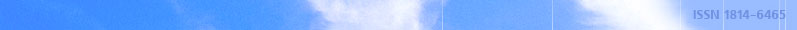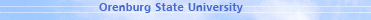december 03, 2021 RU/EN
 Headings of Vestnik Pedagogics Psychology Other

 Search Author Article

# 2001, № 1

D.I. Pastukhov, E.D. Pastukhova DOING A SUM FOR THE EQUATION The article deal nith doing a sum for the equation of the second order derivatives nhen the coefficient is particular. This equation being an operating one for Ux doing particular. This equation being an operating one for doing a sum nith a parallax allows to understand rts structure. Doing a sum е a nith a parallax is determined to exist and to be the only one under indicated conditions.Editor-in-chief Sergey Aleksandrovich MIROSHNIKOV